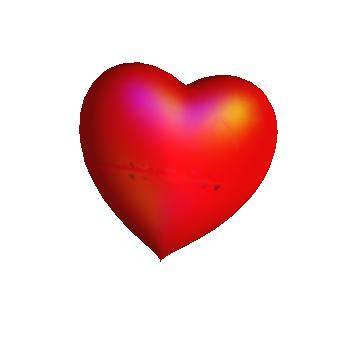# I (x^2 + 9y^2/4 + z^2 – 1)^3 == x^2z^3 + y^2z^3/9 math

Posted by: Gary Ernest Davis on: April 3, 2011

The equation$(x^2 + frac{9y^2}{4} + z^2 - 1)^3 = x^2z^3 + frac{y^2z^3}{9}$ defines a set of points in$xyz$ space that forms a lovely surface, hospital dear to the$(x^2 + frac{9y^2}{4} + z^2 - 1)^3 = x^2z^3 + frac{y^2z^3}{9}$ of all mathematicians:#### Mathematica code

The image above was produced using Mathematica.

Here is the (one line) code:

#### ContourPlot3D[(x^2 + 9*y^2/4 + z^2 – 1)^3 == x^2*z^3 + y^2*z^3/9, {x, -3/2, 3/2}, {y, -3/2, 3/2}, {z, -3/2, 3/2}, Mesh -> None, Boxed -> False, Axes -> False, ContourStyle -> Directive[Red, Opacity[0.8], Specularity[White, 30]]]

x

One can also get a mesh version (not in red) from Wolfram Alpha using the entry